American Journal of Theoretical and Applied Statistics
Volume 4, Issue 3, May 2015, Pages: 85-88

Comparative Study of Efficiency of Integer Programming, Simplex Method and Transportation Method in Linear Programming Problem (LPP)

Department of Mathematics and Statistic, The Polytechnic, Ibadan, Oyo State, Nigeria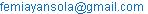(O. Aderemi Ayansola)(I. Favour Oyenuga)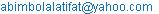(L. Adebisi Abimbola)

Ayansola Olufemi Aderemi, Oyenuga Iyabode Favour, Abimbola Latifat Adebisi. Comparative Study of Efficiency of Integer Programming, Simplex Method and Transportation Method in Linear Programming Problem (LPP). American Journal of Theoretical and Applied Statistics. Vol. 4, No. 3, 2015, pp. 85-88. doi: 10.11648/j.ajtas.20150403.13

Abstract: In this paper, we present a Linear Programming Problem (LPP) to minimize the cost of transportation of NBC, PLC products from three distribution centres to ten depots. Three methods of analysis were considered namely: Integer Programming, simplex method and transportation method via computer packages. The result of the analysis revealed that, the cost of transportation from these distribution centres to all the 10 depots are the same. That is, the optimal cost is N9, 127, 776.

Keywords: Constraints, Algorithm, Simplex Methods, Objective Function, Minimizes

1. Introduction

The purpose of this paper is to give the brief introduction of Linear Programming Problem (LPP) and to look at the three methods say; Integer Linear Programming, Simplex method and Transportation method in solving Linear Programming Problem and find out the method(s) that will gives the minimum cost of transportation. Linear programming (LP) is a tool for solving optimization problems. It is a mathematical modeling "System" which has found widespread uses in providing decision maker with an efficient means for resolving complex operational alternatives. It is applicable to the general category of problems that require the optimization of a linear objective function subject to linear constraints.

In general, Linear Programming is a technique used to determine the best utilization of limited resources to reach a desired objective of either maximizing the benefit (Profit) or minimizing the costs. (Aminu, 1998).

Aremu, M.A (2007) in his paper, titles; A Linear Programming Approach to Profit optimization in a production mixed system, revealed the resources that will maximizes profit to Nigeria Bottling Company, PLC Ilorin Plant in 2003 production.

However, Integer programming techniques have gained a lot of interest recently as they provided state-of-the art methods for predicting RNA secondary structures with Pseudoknops (Poolsap et.al; 2009; Sato et.al; 2011). In our practical life, we come across many business and production industries which have to usually face problems of economics optimization such as cost minimization of non- economic items that are most vital to the existence of their firms. The transportation models are one of these economic optimizations which have their roots in operational management and industrial mathematics as well as since long back 1941. However, the transportation models have applications not only in limited areas of production industries but also worldwide applications in communication networks, planning and scheduling, we refer Ford, L.R and Fulkerson, D.R (1957), Fulkerson, D.R (1961), Garvin, W.W (1960) and Herderson, A and Schlaifer, R (1954).

In various problems of economic optimization, the transportation problem is a logistical problem for organizations especially for manufacturing and transport companies. The difference methods used in solving different versions of transportation models pay a key role in decision-making and process of allocating problem in these organizations, Kirca and Stair (1990).

Furthermore, since the simplex method is an iterative algebraic procedure for solving linear programming problems-transportation models, therefore, keeping in view this feature of the simplest method a few noteworthy previous researchers e.g Arsham, H and Khan, A.B (1989), Arsham, H (1992), Balinski, M.L and Gomory, R.E (1963) among others confined their attention in this direction and developed optimal solution for deterministic transportation models.

2. General Overview of linear Programming Formulation

The general Linear Programming Problem (GLPP) is of the standard form of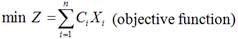(1)

Subject to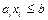(constraints)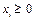(non-negative condition)

In linear systems of equations, we have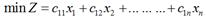s.t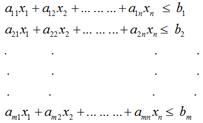Where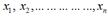are called decision variables,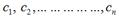are called the cost of variable considered and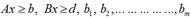are the quantity of materials used.

In this paper, we want to solve the LPP above using the following methods.

Method 1: Integer Programming Method.

The general integer programming problem can be written as

Minimize: CX

Subject to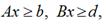(2)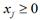and integer, j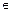I

where b, c and d are vectors, A and B are matrices of conformable dimensions, and the index set I denotes the variables required to be integer. The reason for distinguishing two types of constraints is that the second of these,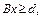is supposed to have special structure.

Integer programming is a valuable tool in operations research, having tremendous potential for applications. Such problems occur quite frequently in business and industry. All assignment and transportation problems are Integer Programming Problem (IPP), the decision variables are either zero or one i.eor 1.

Method 2: Simplex Method

The simplex method is the computational technique used to solve linear programming problem (LPP) involving several number of variables and constraints.

This simplex method is an algorithm for starting at feasible point and by sequence of exchange to other points until a solution point called optimal solution is found.

Method 3: Formulation of Transportation Model

The transportation algorithm is a special designed linear programming for minimizing the total cost of distributing goods from ‘n’ dispatch points to ‘n’ receiving points, provided (i) the number of items to be dispatched from, and the number to be received at, each point is known and (ii) the cost of transportation between each pair of points is known.

In formulation of transportation model, we have;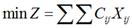Such that: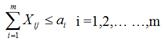(3)

and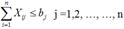with the assumption that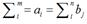3. Data Analysis

The data used for numerical illustration were collected from Nigeria Bottling Company: Asejire, Benin and Ikeja Distribution Centres. The products collected from these three Distribution Centres were distributed to ten (10) depots Viz: Ibadan, Saki, Akure, Sapele, Onitsha, Warri, Ikorodu, Enugu, Abeokuta and Abuja. The above mentioned Distribution Centres and depots were used as a case study for this paper. A computer package was applied for the analysis of the collected data.

From the analysis, it is observed that using the three methods: Integer Linear Programming, Simplex Method and Transportation Method; the result obtained using Microsoft Excel Solver is the same as shown in the table below:

4. Results of Analysis

The values for the decision variables show the optimal quantities to transport over each route. The table above also shows the minimum cost transportation schedule.

Table 1. shows the optimal solution to the Nigerian bottling company transportation problem

 FROM TO UNITS TRANSPORTED COST PER UNIT TOTAL COST Asijere Ibadan 15360 8.30 127488 Ikeja Ibadan 18240 30.00 547200 Asejire Saki 14400 48.50 698400 Asejire Akure 17280 34.40 594432 Benin Sapele 28800 12.50 360000 Ikeja Onitsha 9600 100.80 967680 Asejire Warri 3840 72.60 278784 Benin Warri 14400 24.00 345600 Ikeja Ikorodu 38400 6.00 230400 Benin Enugu 24000 54.00 1296000 Ikeja Abeokuta 23040 24.80 571392 Asejire Abuja 25920 12.00 3110400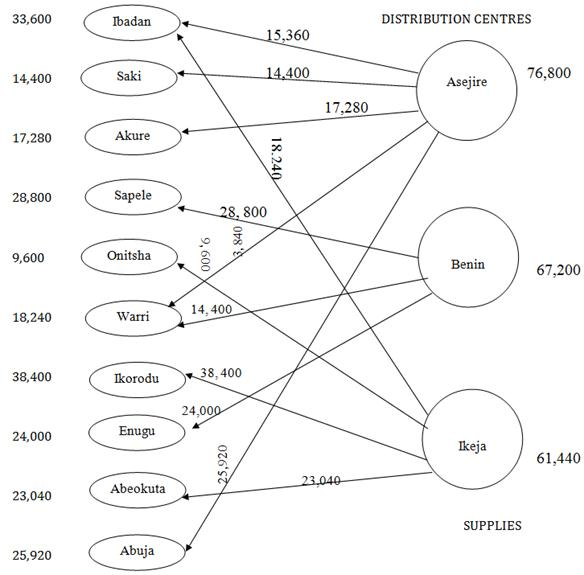Fig. 1. show the summary of the optimal quantities on the network Depots.

5. Conclusion

The results of our analysis revealed that using any of the three methods of obtaining the optimal solution in transportation problem, i.e Simplex Method, Integer Linear Programming Method and Transportation Method yield the same optimal cost. This means that any of the three methods could be used to give optimal cost which would minimizes the total shipping cost with satisfying both demand and supply limits.

Whenever, there is a physical movement of goods from the point of manufacturer to the final consumers through a variety of channels of distribution, there is a need to minimize the cost of transportation so as to increase profit on sales.

References

1. Aminu, Y.A (1998). Operation Research for Science and Management studies.Ijagbo Best Way Printer, Nigeria.
2. Aremu, MA (2007). A Linear Programming Approach to Profit Optimization in a Production Mixed System, Nigerian Journal of Science and Technical Research; Vol. 2, No. 1, pp 30 — 39.
3. Arsham, H (1992). Post optimality analyses of the transportation problem. Journal of the Operational Research Society, Vol. 43, pp. 121-139.
4. Arsham, H and Khan, A.B (1989). A simplex-type algorithm for general transportation problems. An alternative to stepping-stone. Journal Operational Research Society, Vol. 40(6), pp. 581-590.
5. Balinski, M.L and Gomory, R.E (1963). A mutual primal-dual simplex method, Recent Advances in Mathematical Programming (Graves and Wolfe, eds), McGraw-Hill, New York.
6. Ford, L.R and Fulkerson, D.R (1957). A simple algorithm for finding maximal network flows and application to the Hitchcock problem. Canadian Journal of Mathematics, Vol. 9, pp. 210-218.
7. Fulkerson, D.R (1961). An out-of-kilter method for minimal cost flow problems. Journal of the Society for Industrial and Applied Mathematics, Vol. 9, pp. 18-27
8. Garvin, W.W (1960). The distribution of a product from a several sources to numerous localities. Journal of Mathematics Physics. Vol. 20, pp. 224-230.
9. Handy, T (2002). Operations Research- An Introduction (Sixth Edition). Pearson Education Inc.
10. Henderson, A and Schlaifer, R (1954). Mathematical programming: Better information for better decision-making, Harvard Business Review. Vol. 32, pp.73-100.
11. Kirca and Stair (1990). A heuristic for obtaining an initial solution for the transportation problem. Journal of Operational Research Society. Vol. 41(9), pp. 865-867.
12. Kumar, Tapojit (2001). Comparison of Optimization Techniques in large scale Transportations. Journal of undergraduate Research at Minnesota State University, Mankato Vol. 1, Article 10
13. Lucey,, T (1992). Introduction to Quantitative Techniques. London Publication.
14. Marower, M.S and Williamson, E(1970). Teach Yourself Operational Research. English University Press.
15. Poolsap, U. et al (200). Prediction of RNA Secondary Structure with Pseudoknops using integer programming. BMC Bioinformatics, 10 (Suppi. 1), S38.
16. Sato, K. et al (2011). IPknot: fast and accurate prediction of RNA secondary structures with Pseudoknops using integer programming Bioinformatics, 27; i85 — i93.
17. Taha, HA (2003). Operational Research: An Introduction. Prentice Hall Inc(Seventh edition), New York, USA.
18. Winston, W.L (1994). Operation Research; Application and Algorithm (Third Edition). International Thompson Publishing.

 Contents 1. 2. 3. 4. 5.
Article ToolsAbstractPDF(204K)